# Fundamental Quantities

Before proceeding further, it is helpful to define a few fundamental quantities.

Of course, quasi-neutrality demands that(2.16)

We can estimate typical particle speeds in terms of the so-called thermal speed ,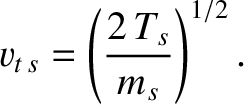(2.17)

The typical gyro-radius of a charged particle gyrating in the magnetic field of a tokamak is given by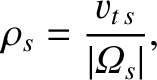(2.18)

where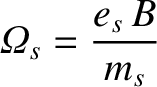(2.19)

is the gyro-frequency associated with the gyration . (Note that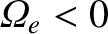, indicating that electrons gyrate around magnetic field-lines in the opposite direction to ions.)

The electron-ion and ion-ion collision times are written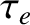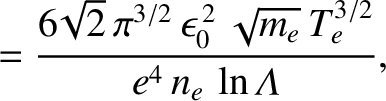(2.20)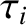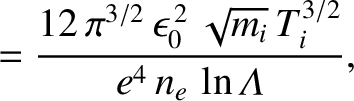(2.21)

respectively . Here,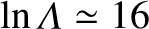is the Coulomb logarithm . Note thatis the typical time required for the cumulative effect of electron-ion collisions to deviate the path of an electron through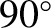. Likewise,is the typical time required for the cumulative effect of ion-ion collisions to deviate the path of an ion through.

The electron and ion collision frequencies are simply the inverses of the correspondingcollision times: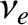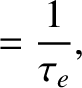(2.22)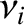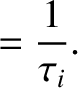(2.23)

Finally, the mean-free-paths between collisions (i.e.,scattering events) for electrons and ions are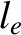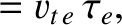(2.24)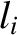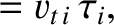(2.25)

respectively.

Table 2.1 gives estimates for some of the fundamental plasma parameters defined in this section in a low-field and a high-field tokamak fusion reactor. Here, use has been made of the data shown in Table 1.2. It has also been assumed that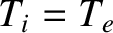, for the sake of simplicity.

Table: 2.1 Fundamental plasma parameters in a low-field and a high-field tokamak reactor. Here,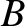is the toroidal magnetic field-strength,the plasma major radius,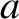the plasma minor radius,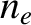the electron number density,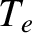the electron temperature,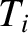the ion temperature,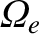the electron gyro-frequency,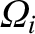the ion gyro-frequency,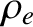the electron gyro-radius,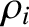the ion gyro-radius,the electron collision time,the ion collision time,the electron mean-free-path, andthe ion mean-free-path.
 Low-Field High-Field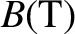5.0 12.0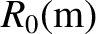7.6 3.2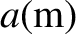2.5 1.1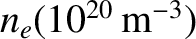0.89 5.1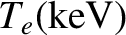7.0 7.0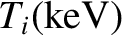7.0 7.0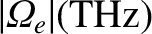0.88 2.1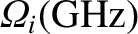0.19 0.46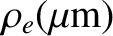56 24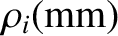3.8 1.6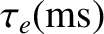0.14 0.025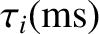13.6 2.36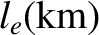7.0 1.2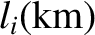10 1.7